# Integers + divisibility - math problems

#### Number of problems found: 23

• What is 16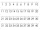What is the sum of three consecutive even integers such that six more than twice the second is 2/3 of the first increased by 3/2 of the third?
• AND-NOT-ANDIf P is the set of multiples of 2, Q is the set of multiples of 3, and R is the set of multiples of 7, which of the following integers will be in P and Q but not in R? A=−54 B=−50 C=42 D=100 E=252
• Sum of odd numbersFind the sum of all odd integers from 13 to 781.
• Integer cubeThe length of the cube edge is an integer. Its volume is in cm3 a five-digit number divisible by 1331. What is the length of the edge of this cube.
• Three-digit integersHow many three-digit natural numbers exist that do not contain zero and are divisible by five?
• DozenWhat is the product of 26 and 5? Write the answer in an Arabic numeral. Add up the digits. How many of this is in a dozen? Divide #114 by this
• Year 2018The product of the three positive numbers is 2018. What are the numbers?
• The sum 2The sum of five consecutive even integers is 150. Find the largest of the five integers. A.28 B.30 C.34 D.54 Show your solution and explain your answer.
• Candy and boxesWe have some candy and empty boxes. When we put candies in boxes of ten, there will be 2 candies and 8 empty boxes left. When of eight, there will be 6 candies and 3 boxes left. How many candy and empty boxes left when we put candies in boxes of nine?
• SchoolLess than 500 pupils attend school. When it is sorted into pairs, one pupil remains. Similarly, when sorted into 3, 4, 5 and 6 members team one remains. Sorted to seven members teams, no left behind. How many pupils are attending this school?
• TogetherIf 8 men, 10 women, 16 children collects ₹1024 in 4 days, how many days will be required for 6 men, 5 women and 4 boys to collect ₹768? (₹ is Indian Rupee)
• One hundred stamps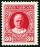A hundred letter stamps cost a hundred crowns. Its costs are four levels - twenty tenths , one crown, two-crown and five-crown. How many are each type of stamps? How many does the problem have solutions?
• Digit sumDetermine for how many integers greater than 900 and less than 1,001 has digit sum digit of the digit sum number 1.
• Modulo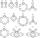Find x in modulo equation: 47x = 4 (mod 9) Hint - read as: what number 47x divided by 9 (modulo 9) give remainder 4 .
• Clotheslines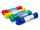Mommy needs 6 clothesline 360 cm long. Clotheslines is sold in the length of eight meters. How many of these clotheslines must buy mom if any does not want to be connected? How much mommy pay for the clotheslines when 1 piece costs 24kč?
• DivisibilityWrite all the integers x divisible by seven and eight simultaneously, for which the following applies: 100 < x < 200.
• Snowman 2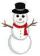On the medal, which has the shape of a circle with a diameter 18 cm is sketched snowman so that the following requirements are met: 1. snowman is composed of three circles, 2. space over snowman is the same as under it, 3. diameters of all circles express
• Snowman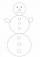In a circle with a diameter 50 cm are drawn 3 circles /as a snowman/ where: its diameters are integers, each larger circle diameter is 3 cm larger than the diameter of the previous circle. Determine snowman height if we wish highest snowman.
• Package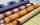The package has no more than 66 m of cloth. If we just cut it all on the blouses or all on dresses, no cloth left remain. On the one blouse consumes 1.3 m of cloth and on one dress 5 m. Determine the amount of the cloth in the package.
• Class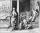When Pythagoras asked how many students attend the school, he said: "Half of the students studying mathematics, 1/4 music, seventh silent, and there are three girls at school." How many students had Pythagoras at school?

Do you have an exciting math question or word problem that you can't solve? Ask a question or post a math problem, and we can try to solve it.

We will send a solution to your e-mail address. Solved examples are also published here. Please enter the e-mail correctly and check whether you don't have a full mailbox.

Integers - math problems. Divisibility - math problems.